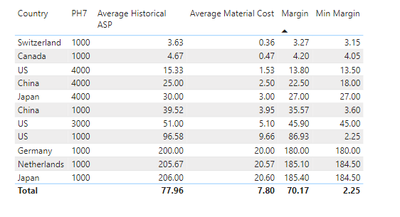cancel
Showing results for
Did you mean:Frequent Visitor

## MINX of Measure not behaving as expected

I have a slicer to select a Country, and a table with 3 measures based on this slicer. The three measures are: Average Historical ASP, Average Material Cost, and Margin. I am trying to add a 4th measure which finds the minimum value from all values in the Margin column.

The formula I am currently using is:

Min Margin = MINX('Historical ASP', [Margin])I would expect that with all Country values selected, it should show 3.27 as Min Margin for all rows in the above. Where am I going wrong?

1 ACCEPTED SOLUTIONSuper User

@colsen12 Try:

``````Min Margin =
VAR __Table = SUMMARIZE(ALL('Historical ASP'),[Country],"__Margin",[Margin])
VAR __Result = MINX(__Table,[__Margin])
RETURN
__Result``````

Become an expert!: Enterprise DNA
External Tools: MSHGQM
Latest book!:
Mastering Power BI 2nd EditionDAX is easy, CALCULATE makes DAX hard...
4 REPLIES 4Community Champion

Seems on the wrong granularity,  try like:

``````Min Margin =
MINX(
SUMMARIZE(
'Historical ASP',
'Historical ASP'[Country],
'Historical ASP'[PH7]
),
[Margin]
)``````Super User

@colsen12 Try:

``````Min Margin =
VAR __Table = SUMMARIZE(ALL('Historical ASP'),[Country],"__Margin",[Margin])
VAR __Result = MINX(__Table,[__Margin])
RETURN
__Result``````

Become an expert!: Enterprise DNA
External Tools: MSHGQM
Latest book!:
Mastering Power BI 2nd EditionDAX is easy, CALCULATE makes DAX hard...Frequent Visitor

@Greg_Deckler Thanks that works. Was wondering, if I wanted to measure MINX only for selected Country fields from the slicer, where do I add that in?Super User